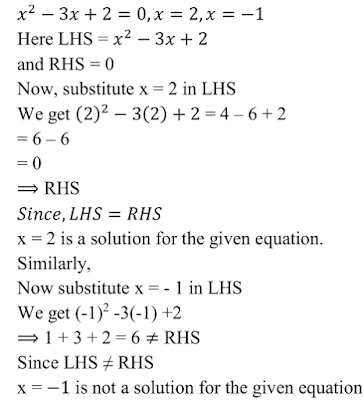## 1. Which of the following are quadratic equations ?Solution2. In each of the following , determine whether the given values are solutions of the given equation or not :Solution (i)(ii)(iii)(iv)(v)(vi)(vii)3. In each of the following , find the value of k for which the given value is a solution of the given equation :Solution (i)(ii)(iii)(iv)4. Determine if, 3 is a root of the equation given below:Solution5. If x = 2/3 and x = -3 are the roots of the equation ax2 + 7x + b = 0, find the values of a and b .    Solution We know that x = -2/3 and x = -3 are the roots of the equation ax2 + 7x + b. When we the value of x in this equation, we will get the result as 0. Putting x = -2/3 a(2/3)2 + 7(2/3) + b = 0 ⇒ 4/9 a +14/3 + b =0 ⇒ 4/9 a  + b = -14/3 --- (i) Putting x = -3 a(-3)2 + 7(-3) + b = 0 ⇒ 9a - 21 + b =0 ⇒ 9a  + b = 21 --- (ii) Subtracting (i)  from (ii), we get 9a  + b - (4/9 a  + b) = 21 + 14/3 ⇒ 9a  + b - 4/9 a  -b = 77/3 ⇒ 77/9 a = 49/3 ⇒  a = 77/3 × 9/77 ⇒  a = 3 Now, putting a =3 in equation (ii), 9×3 + b = 21 ⇒ 27 + b = 21 ⇒  b = 21 - 27 ⇒  b = -6 Thus, a = 3 and b = -6

X
Free Study Rankers App Download Now